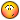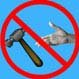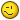# error Trapping??

## Recommended PostsHere is a Lisp routine that draws a text box around text,mtext,or dimensions i modified it to draw the box and allow the user to trim inside of the box then the box goes away but if you hit the escape button at any point then all of the osnap settings turn off. Can anyone help with the error trap on this lisp? Ive tried but no full success yet. Thanks

~Psalms 30:5~

##### Share on other sites```(defun c:TB ( )(c:Text-Box));Shortcut
(defun c:Text-Box (/ Cnt# EntName^ Osmode# Pt PtsList@ SS& ss ln1 ln2 eln1 eln2 pln1 pln2 ln1p1 ln1p2 ln2p1 ln2p2
p1 p2 p3 p4 cmd osm)
(setq Osmode# (getvar "OSMODE"))
(princ "\nSelect Text, Mtext or Dimension for Text Box")
(if (setq SS& (ssget '((-4 . "<OR")(0 . "TEXT")(0 . "MTEXT")(0 . "DIMENSION")(-4 . "OR>"))))
(progn
(command "UNDO" "BEGIN")
(setvar "osmode" 4)
(setq Cnt# 0)
(repeat (sslength SS&)
(setq EntName^ (ssname SS& Cnt#))
(setq PtsList@ (append (Text-Box EntName^) (list "C")))
(setq Cnt# (+ 4 Cnt#))
(command "PLINE" (foreach Pt PtsList@  (command Pt) ))
(command "_offset" "_erase" "_yes" 3.75 (entlast) "0,0,0" "exit")

);repeat
(setvar "OSMODE" Osmode#)
(command "_trim" "_last" "" "_crossing"(while(> (getvar "cmdactive")0)(command pause) ptslist@)"" "_erase" "_previous"  "")
(command "_offset" "e" "no" "" "_EXIT")
(command "UNDO" "END")
(setvar "OSMODE" Osmode#)
(redraw)
);progn
(princ "\nNo Text, Mtext or Dimension selected.")
)
(princ)
);defun c:Text-Box
;-------------------------------------------------------------------------------
; Text-Box - Function for Text, Mtext and Dimension entities
; Arguments: 1
;   Entity^ = Entity name of the Text, Mtext or Dimension to use
; Returns: A list of the four corners of the Text Box
;-------------------------------------------------------------------------------
(defun Text-Box (Entity^ / Ang~ AngEntity~ Corners: EntList@ EntNext^ EntType\$
First List@ MovePt NewPts@ Pt Return@ Textboxes@ X X1 X3 Y Y1 Y3 Zero)
;-----------------------------------------------------------------------------
; Corners: - Calculates the four corners of the Text Box
;-----------------------------------------------------------------------------
(defun Corners: (Entity^ / Ang~ Corners@ Dist~ EntList@ Ins Pt Pt1 Pt2 Pt3 Pt4)
(setq EntList@ (entget Entity^)
Corners@ (textbox EntList@)
Ang~ (cdr (assoc 50 EntList@))
Ins (cdr (assoc 10 EntList@))
Pt (mapcar '+ (car Corners@) Ins)
Pt1 (polar Ins (+ Ang~ (angle Ins Pt)) (distance Ins Pt))
Pt (mapcar '+ (cadr Corners@) Ins)
Pt3 (polar Ins (+ Ang~ (angle Ins Pt)) (distance Ins Pt))
Dist~ (* (distance (car Corners@) (cadr Corners@)) (cos (- (angle Pt1 Pt3) Ang~)))
Pt2 (polar Pt1 Ang~ Dist~)
Pt4 (polar Pt3 Ang~ (- Dist~))

);setq
(list Pt1 Pt2 Pt3 Pt4)
);defun Corners:
;-----------------------------------------------------------------------------
(setq EntList@ (entget Entity^)
EntType\$ (cdr (assoc 0 EntList@))
);setq
(cond
((= EntType\$ "TEXT")
(setq Return@ (Corners: Entity^))
);case
((or (= EntType\$ "MTEXT")(= EntType\$ "DIMENSION"))
(command "UNDO" "MARK")
(setq EntNext^ (entlast))
(command "EXPLODE" Entity^)
(if (= EntType\$ "DIMENSION")
(command "EXPLODE" (entlast))
);if
(while (setq EntNext^ (entnext EntNext^))
(if (= "TEXT" (cdr (assoc 0 (entget EntNext^))))
(setq Textboxes@ (append Textboxes@ (list (Text-Box EntNext^))))
);if
);while
(command "UNDO" "BACK")
(setq AngEntity~ (angle (nth 0 (nth 0 [email="Textboxes@))(nth"]Textboxes@))(nth[/email] 1 (nth 0 Textboxes@)))
Zero (list 0 0)
First t
);setq
(foreach List@ Textboxes@
(foreach Pt List@
(setq X (car Pt) Y (cadr Pt))
(if First
(setq First nil X1 X Y1 Y)
);if
(if (< X X1)(setq X1 X))
(if (< Y Y1)(setq Y1 Y))
);foreach
);foreach
(if (or (< X1 0)(< Y1 0))
(progn
(cond
((and (< X1 0)(< Y1 0))(setq MovePt (list X1 Y1)))
((< X1 0)(setq MovePt (list X1 0)))
((< Y1 0)(setq MovePt (list 0 Y1)))
(setq x1 (+ 1))
(setq y1 (+ 1))
);cond
(command "UCS" "M" MovePt)
);progn
);if
(setq First t)
(foreach List@ Textboxes@
(foreach Pt List@
(setq Ang~ (- (angle Zero Pt) AngEntity~))
(setq Pt (polar Zero Ang~ (distance Zero Pt)))
(setq X (car Pt) Y (cadr Pt))
(if First
(setq First nil X1 X X3 X Y1 Y Y3 Y)
);if
(if (< X X1)(setq X1 X))
(if (< Y Y1)(setq Y1 Y))
(if (> X X3)(setq X3 X))
(if (> Y Y3)(setq Y3 Y))
);foreach
);foreach
(command "UCS" "W")
(setq NewPts@ (list (list X1 Y1)(list X3 Y1)(list X3 Y3)(list X1 Y3)))
(foreach Pt NewPts@
(setq Ang~ (+ (angle Zero Pt) AngEntity~))
(setq Pt (polar Zero Ang~ (distance Zero Pt)))
(setq Return@ (append Return@ (list Pt)))
);foreach
);case
)
Return@
);defun Text-Box
;-------------------------------------------------------------------------------
(princ)

```

##### Share on other sitesby far the best error handler ive seen to date would have to be from a friend named Evgeniy.

Post by: *ElpanovEvgeniy*

------------------------------------------------------------------------

In the beginning of function I establish a list of the necessary environment variables,

list variable always miscellaneous:

Code:

```(SETQ
ERROR-LST-
'("AUTOSNAP" "OSMODE" "APERTURE" "HPSPACE" "HPASSOC" "MIRRTEXT" "AUPREC" "LUPREC"
"DIMZIN" "cecolor"
)
ERROR-LST- (mapcar (function (lambda (a) (list 'setvar a (getvar a)))) ERROR-LST-)
) ;_  SETQ```

Function *error*

Code:

`(defun *error* (msg) (MAPCAR 'eval ERROR-LST-))`

It is a universal *error* function :-)

Give it a try:

(*error* "")

##### Share on other sitesSe7en, just about beat me to it:

```(defun c:tb () (c:text-box))
(defun c:text-box  (/ *error* vlst ovars cnt# entname^ osmode# pt ptslist@ ss& ss ln1
ln2 eln1 eln2 pln1 pln2 ln1p1 ln1p2 ln2p1
ln2p2 p1 p2 p3 p4 cmd osm)

(defun *error*  (msg)
(if    ovars
(mapcar 'setvar vlst ovars))
(princ))

(setq    vlst  '("OSMODE")  ; <<--- List changed variables here
ovars (mapcar 'getvar vlst))

(princ "\nSelect Text, Mtext or Dimension for Text Box")
(setq osmode# (getvar "OSMODE"))
(if (setq ss& (ssget '((-4 . "<OR") (0 . "TEXT") (0 . "MTEXT") (0 . "DIMENSION") (-4 . "OR>"))))
(progn
(command "UNDO" "BEGIN")
(setvar "osmode" 4)
(setq cnt# 0)
(repeat (sslength ss&)
(setq entname^ (ssname ss& cnt#))
(setq ptslist@ (append (text-box entname^) (list "C")))
(setq cnt# (+ 4 cnt#))
(command "PLINE" (foreach pt ptslist@ (command pt)))
(command "_offset" "_erase" "_yes" 3.75 (entlast) "0,0,0" "exit")

) ;repeat
(setvar "OSMODE" osmode#)
(command "_trim"
"_last"
""
"_crossing"
(while (> (getvar "cmdactive") 0) (command pause) ptslist@)
""
"_erase"
"_previous"
"")
(command "_offset" "e" "no" "" "_EXIT")
(command "UNDO" "END")
(setvar "OSMODE" osmode#)
(redraw)
) ;progn
(princ "\nNo Text, Mtext or Dimension selected.")
)
(mapcar 'setvar vlst ovars)
(princ)
) ;defun c:Text-Box

;-------------------------------------------------------------------------------
; Text-Box - Function for Text, Mtext and Dimension entities
; Arguments: 1
;   Entity^ = Entity name of the Text, Mtext or Dimension to use
; Returns: A list of the four corners of the Text Box
;-------------------------------------------------------------------------------
(defun text-box     (entity^   /          ang~    angentity~        corners:  entlist@    entnext^  enttype\$  first
list@        movept    newpts@    pt      return@   textboxes@        x      x1        x3
y        y1          y3    zero)
;-----------------------------------------------------------------------------
; Corners: - Calculates the four corners of the Text Box
;-----------------------------------------------------------------------------
(defun corners:  (entity^ / ang~ corners@ dist~ entlist@ ins pt pt1 pt2 pt3 pt4)
(setq entlist@ (entget entity^)
corners@ (textbox entlist@)
ang~       (cdr (assoc 50 entlist@))
ins       (cdr (assoc 10 entlist@))
pt       (mapcar '+ (car corners@) ins)
pt1       (polar ins (+ ang~ (angle ins pt)) (distance ins pt))
pt       (mapcar '+ (cadr corners@) ins)
pt3       (polar ins (+ ang~ (angle ins pt)) (distance ins pt))
dist~       (* (distance (car corners@) (cadr corners@)) (cos (- (angle pt1 pt3) ang~)))
pt2       (polar pt1 ang~ dist~)
pt4       (polar pt3 ang~ (- dist~))

) ;setq
(list pt1 pt2 pt3 pt4)
) ;defun Corners:
;-----------------------------------------------------------------------------
(setq    entlist@ (entget entity^)
enttype\$ (cdr (assoc 0 entlist@))
) ;setq
(cond
((= enttype\$ "TEXT")
(setq return@ (corners: entity^))
) ;case
((or (= enttype\$ "MTEXT") (= enttype\$ "DIMENSION"))
(command "UNDO" "MARK")
(setq entnext^ (entlast))
(command "EXPLODE" entity^)
(if (= enttype\$ "DIMENSION")
(command "EXPLODE" (entlast))
) ;if
(while (setq entnext^ (entnext entnext^))
(if (= "TEXT" (cdr (assoc 0 (entget entnext^))))
(setq textboxes@ (append textboxes@ (list (text-box entnext^))))
) ;if
) ;while
(command "UNDO" "BACK")
(setq angentity~ (angle (nth 0 (nth 0 textboxes@)) (nth 1 (nth 0 textboxes@)))
zero          (list 0 0)
first      t
) ;setq
(foreach list@  textboxes@
(foreach    pt  list@
(setq x (car pt)
(if first
(setq first nil
x1    x
y1    y)
) ;if
(if (< x x1)
(setq x1 x))
(if (< y y1)
(setq y1 y))
) ;foreach
) ;foreach
(if (or (< x1 0) (< y1 0))
(progn
(cond
((and (< x1 0) (< y1 0)) (setq movept (list x1 y1)))
((< x1 0) (setq movept (list x1 0)))
((< y1 0) (setq movept (list 0 y1)))
(setq x1 (+ 1))
(setq y1 (+ 1))
) ;cond
(command "UCS" "M" movept)
) ;progn
) ;if
(setq first t)
(foreach list@  textboxes@
(foreach    pt  list@
(setq ang~ (- (angle zero pt) angentity~))
(setq pt (polar zero ang~ (distance zero pt)))
(setq x (car pt)
(if first
(setq first nil
x1    x
x3    x
y1    y
y3    y)
) ;if
(if (< x x1)
(setq x1 x))
(if (< y y1)
(setq y1 y))
(if (> x x3)
(setq x3 x))
(if (> y y3)
(setq y3 y))
) ;foreach
) ;foreach
(command "UCS" "W")
(setq newpts@ (list (list x1 y1) (list x3 y1) (list x3 y3) (list x1 y3)))
(foreach pt  newpts@
(setq ang~ (+ (angle zero pt) angentity~))
(setq pt (polar zero ang~ (distance zero pt)))
(setq return@ (append return@ (list pt)))
) ;foreach
) ;case
)
return@
) ;defun Text-Box
;-------------------------------------------------------------------------------
(princ)
```

^^ not quite as elegant##### Share on other sites> ... not quite as elegant...

Oh don't feel bad at! i was blown away when i first saw his solution (I almost fell out of my chair as a matter of fact!). It's quite amazing isnt it? Besides, looks like you had the same idea anyways.

##### Share on other sitesthis is the *error* handler i keep in my template. you are more than welcome to this. i went ahead and added the reset of the osmode and an undo end. if using this type of *error* handler, you MUST localize it (place it in the main routine and add *error* to your local variables.

```;;;error handler

(defun *error* (msg)
(and Osmode# (setvar "osmode" Osmode#))
(command "_.undo" "_e")
(if
(not
(member
msg
'("console break" "Function cancelled" "quit / exit abort")
) ;_ member
) ;_ not
(princ (strcat "\nError: " msg))
) ;_ if
) ;_ defun```

##### Share on other sitesLooks like he's thinking about it in a "script" kind of fashion - creating a list of coding that can be evaulated at will.

Nice idea##### Share on other sitesif using this type of *error* handler, you MUST localize it (place it in the main routine and add *error* to your local variables.

Good point - I have had many a discussion about localising error handlers as opposed to

```(setq olderr *error* *error* newerr)
(setq *error* olderr)
etc etc

```

I'm not sure that there is a "right" way to do it, but I much prefer to localise the *error* after redefining it.

##### Share on other siteswell crap, i guess everyone beat me to the punch.

john, once again, you prove to be my hero.

yuck, i hate the way pasted in code looks when i'm on my mac.

##### Share on other sitesJust an alternative:

Something I picked up from David Bethel:

```
;++++++++++++ Set Modes & Error ++++++++++++++++++++++++++++++++++
(defun nw_smd ()
(SetUndo)
(setq oldlay  (getvar "CLAYER")
olderr  *error*
*error* (lambda (e)
(while (> (getvar "CMDACTIVE") 0)
(command)
) ;_  end while
(and (/= e "quit / exit abort")
(princ (strcat "\nError: *** " e " *** "))
) ;_  end and
(and (= (logand (getvar "UNDOCTL")  8)
(command "_.UNDO" "_END" "_.U")
) ;_  end and
(nw_rmd)
) ;_  end lambda
nw_var  '(("CMDECHO" . 0)
("MACROTRACE" . 0)
("OSMODE" . 0)
("SORTENTS" . 119)
("MODEMACRO" . ".")
("LUPREC" . 2)
("BLIPMODE" . 0)
("EXPERT" . 0)
("SNAPMODE" . 1)
("PLINEWID" . 0)
("ORTHOMODE" . 1)
("GRIDMODE" . 0)
("ELEVATION" . 0)
("THICKNESS" . 0)
("FILEDIA" . 0)
("FILLMODE" . 0)
("SPLFRAME" . 0)
("UNITMODE" . 0)
("TEXTEVAL" . 0)
("ATTDIA" . 0)
("AFLAGS" . 0)
("ATTREQ" . 1)
("ATTMODE" . 1)
("UCSICON" . 1)
("HIGHLIGHT" . 1)
("REGENMODE" . 1)
("COORDS" . 2)
("DRAGMODE" . 2)
("DIMZIN" . 1)
("PDMODE" . 0)
("CECOLOR" . "BYLAYER")
("CELTYPE" . "BYLAYER")
)
) ;_  end setq
(foreach v nw_var
(and (getvar (car v))
(setq nw_rst (cons (cons (car v) (getvar (car v))) nw_rst))
(setvar (car v) (cdr v))
) ;_  end and
) ;_  end foreach
(princ (strcat (getvar "PLATFORM") " Release " (ver)))
(princ)
) ;_  end defun

(PDot) ;++++++++++++ Return Modes & Error +++++++++++++++++++++++++++++++
(defun nw_rmd ()
(SetLayer oldlay)
(setq *error* olderr)
(foreach v nw_rst (setvar (car v) (cdr v)))
(command "_.UNDO" "_END")
(prin1)
) ;_  end defun

(PDot) ;++++++++++++ Set And Start An Undo Group ++++++++++++++++++++++++
(defun SetUndo ()
(and (zerop (getvar "UNDOCTL"))
(command "_.UNDO" "_ALL")
) ;_  end and
(and (= (logand (getvar "UNDOCTL") 2) 2)
(command "_.UNDO" "_CONTROL" "_ALL")
) ;_  end and
(and (= (logand (getvar "UNDOCTL")  8)
(command "_.UNDO" "_END")
) ;_  end and
(command "_.UNDO" "_GROUP")
) ;_  end defun

(PDot) ;++++++++++++ Make Layer Current +++++++++++++++++++++++++++++++++
(defun SetLayer    (name / ldef flag)
(command "_.LAYER")
(if    (not (tblsearch "LAYER" name))
(command "_Make" name)
(progn
(setq ldef (tblsearch "LAYER" name)
flag (cdr (assoc 70 ldef))
) ;_  end setq
(and (= (logand flag 1) 1)
(command "_Thaw" name)
) ;_  end and
(and (minusp (cdr (assoc 62 ldef)))
(command "_On" name)
) ;_  end and
(and (= (logand flag 4) 4)
(command "_Unlock" name)
) ;_  end and
(and (= (logand flag 16) 16)
(princ "\nCannot Set To XRef Dependent Layer")
(quit)
) ;_  end and
(command "_Set" name)
) ;_  end progn
) ;_  end if
(command "")
name
) ;_  end defun

;************ Main Program ***************************************
(defun nw_ (/ olderr oldlay nw_var nw_rst)
(nw_smd)

(nw_rmd)
) ;_  end defun

(defun C:NW () (nw_))
(if nw_
) ;_  end if
(prin1)
```

Most Complicated Error Template I have seen...

##### Share on other sitesComplex? *Pthhht!* I have one that i based on one from Vladimir Nesterovsky and ElpanovEvgeniy's It was quite big.

alanjt, I guess i had no idea you were on this site; hello.

##### Share on other sitesComplex? *Pthhht!* I have one that i based on one from Vladimir Nesterovsky and ElpanovEvgeniy's It was quite big.

alanjt, I guess i had no idea you were on this site; hello.

nice, none the less.

yeah, i get aroundi didn't know you were on here either.

##### Share on other sitesWell guys It looks like there are more ways to use the error trap than i thought. I appreciate the solution but more appreciate the info!!

I added one line to this and it works like a charm. Before if you hit escape the box would stay but this not only restores the vars but erases the box too. So thanks again

```;;;error handler
(defun *error* (msg)
(and Osmode# (setvar "osmode" Osmode#))
[color=red] (and(command "_erase" (entlast)""))[/color]
(command "_.undo" "_e")
(if
(not
(member
msg
'("console break" "Function cancelled" "quit / exit abort")
) ;_ member
) ;_ not
```

##### Share on other sitesI wouldn't use "entlast" if I were you - if you set the box entity to some variable, test for the box's creation first:

i.e.

```(if box (entdel box))
```

Otherwise, this could cause an error in itself if the box is not made before the user hits escape.

##### Share on other sitesBut it is also More simple and Just as Effective to just have

`[color=#ff0000](and(command "_erase" "previous" ""))[/color]`

is it not?

##### Share on other sitesBut it is also More simple and Just as Effective to just have

`[color=#ff0000](and(command "_erase" "previous" ""))[/color]`

is it not?

I just try to steer clear of "command" calls thats all.

##### Share on other sitesBut it is also More simple and Just as Effective to just have

`[color=#ff0000](and(command "_erase" "previous" ""))[/color]`

is it not?

You must bear in mind that your box may not be drawn when the user hits escape, so this may erase any previous entity drawn before your box.

##### Share on other sitesYes but now with the erase previous it will not erase any entity thats was made before the command was envoked. Even if the box was not drawn first.

##### Share on other sitesIn the blink of an eye.

```( (lambda ( / ent ent-handle )
(setq ent (entget (car (entsel)))
ent-handle (cdr (assoc 5 ent)))
;; get the entity and store the handle
;; using the handle we can obtain the ename of the entity
(entdel (handent ent-handle))
;; delete the entity

(entdel (handent ent-handle))
;; put it back

(princ)
)
)```

##### Share on other sitesI haven't tested it but does that not require the user to pick an entity, with the use of "entsel"?

## Join the conversation

You can post now and register later. If you have an account, sign in now to post with your account.
Note: Your post will require moderator approval before it will be visible.×   Pasted as rich text.   Paste as plain text instead

Only 75 emoji are allowed.